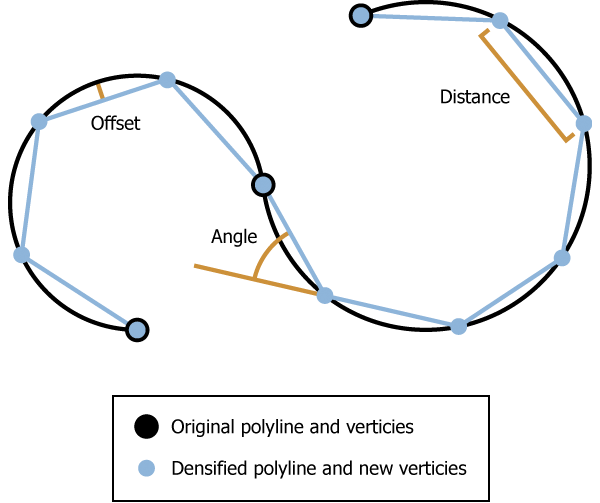# Densify (Editing)

## Summary

Adds vertices along line or polygon features. Also replaces curve segments (Bezier, circular arcs, and elliptical arcs) with line segments.

## Illustration## Usage

##### Caution:

This tool modifies the input data. See Tools that do not create output datasets for more information and strategies to avoid undesired data changes.

• Straight line segments are densified by the Distance parameter. Curve segments are simplified through densification by the Distance, Maximum Deflection Angle, or Maximum Offset Deviation parameter.

• Densification is done segment by segment.

• Only one densification method can be selected each time Densify is executed.

• The spatial reference of the data is very important to the result generated by this tool. Densify the data in an appropriate coordinate system to maintain the correct shape of the features.

• For each vertex of the original feature, including the start and end points, there will be a coincident vertex in the resulting feature.

• Maximum Vertex Count controls the maximum number of vertices in each densified output segment. If a densify operation requires more than this value, the segments will be adjusted to ensure it does not exceed this vertex count (+/- 1). If an invalid value is entered (0 or less), there will be no limit applied to linear segments, and the tool will default to 12000 for curve segments.

Setting Maximum Vertex Count will cause the set value to be used for the maximum vertex count for both linear and curve segments.

##### Note:
The larger the value used, the greater the chance of a feature growing very large. Overly large features may cause a negative performance impact. If an extremely small value is used, the output feature may be degenerate, resulting in a null geometry. Extremely small values may also result in the creation of features that return unexpected results in subsequent analyses.

• When densifying by Maximum Offset Deviation, if the input geometry contains circular arcs, an upper limit of the offset will be enforced so that the angle between two consecutive line segments in the output will not exceed 10 degrees. This angle can be exceeded if you densify by Maximum Deflection Angle.

## Syntax

`Densify(in_features, {densification_method}, {distance}, {max_deviation}, {max_angle}, {max_vertex_per_segment})`
 Parameter Explanation Data Type in_features The polygon or line feature class to be densified. Feature Layer densification_method(Optional) Specifies the feature densification method to be used.DISTANCE —The distance parameter value will be applied to curves the same as it is to straight lines. This is the default. OFFSET —The max_deviation parameter value will be applied to curves. ANGLE —The max_angle parameter value will be applied to curves. String distance(Optional) The maximum linear distance between vertices. This distance will always be applied to line segments and to simplify curves. The default value is a function of the data's X,Y tolerance. Linear Unit max_deviation(Optional) The maximum distance the output segment will be from the original. This parameter only affects curves. The default value is a function of the data's X,Y tolerance. Linear Unit max_angle(Optional) The maximum angle the output geometry can be from the input geometry. The valid range is 0 to 90. The default value is 10. This parameter only affects curves. Double max_vertex_per_segment(Optional) The maximum vertex count allowed per segment. If no value or an invalid value (0 or less) is entered, there will be no vertex limit for linear segments, and curve segments will have a default of 12000. Long

#### Derived Output

 Name Explanation Data Type out_feature_class The densified input features. Feature Class

## Code sample

Densify example 1 (Python window)

The following Python window script demonstrates how to use the Densify function in immediate mode.

``````import arcpy
arcpy.Densify_edit("C:/data.gdb/lines", "ANGLE", "", "", "0.75")``````
Densify example 2 (stand-alone script)

The stand-alone script below shows the Densify function as part of a workflow that also uses the Snap editing tool.

``````# Name: Snap.py
# Description: Snap climate regions boundary to vegetation layer
#              boundary to ensure common boundary is coincident

# import system modules
import arcpy

# Set environment settings
arcpy.env.workspace = "C:/data"

# Make backup copy of climate regions feature class,
# since modification with the Editing tools below is permanent.
climateBackup = "backups/climate.shp"
arcpy.CopyFeatures_management('climate.shp', climateBackup)

# Densify climate regions feature class to make sure there are enough vertices
# to match detail of vegetation layer when layers are snapped.
arcpy.Densify_edit('climate.shp', "DISTANCE", "10 Feet")

# Snap climate regions feature class to vegetation layer vertices and edge.
# First, snap climate region vertices to the nearest vegetation vertex within
# 30 Feet. Second, snap climate region vertices to the nearest vegetation edge
# within 20 Feet.
snapEnv1 = ["Habitat_Analysis.gdb/vegtype", "VERTEX", "30 Feet"]
snapEnv2 = ["Habitat_Analysis.gdb/vegtype", "EDGE",   "20 Feet"]
arcpy.Snap_edit('climate.shp', [snapEnv1, snapEnv2])``````

## Licensing information

• Basic: No
• Standard: Yes